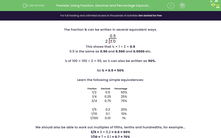# Using Fraction, Decimal and Percentage Equivalences

In this worksheet, students use equivalences between simple fractions, decimals and percentages, including in different contexts.Key stage:  KS 2

Curriculum topic:   Number: Fractions, Decimals and Percentages

Curriculum subtopic:   Know Fraction-Decimal-Percentage Equivalents

Difficulty level:#### Worksheet Overview

The fraction ½ can be written in several equivalent ways.This shows that ½ = 1 ÷ 2 = 0.5

0.5 is the same as 0.50 and 0.500 and 0.5000 etc.

½ of 100 = 100 ÷ 2 = 50, so ½ can also be written as 50%.

So ½ = 0.5 = 50%

Learn the following simple equivalences:

Fraction Decimal Percentage
1/2 0.5 50%
1/4 0.25 25%
3/4 0.75 75%

1/5 0.2 20%
1/10 0.1 10%
1/100 0.01 1%

We should also be able to work out multiples of fifths, tenths and hundredths, for example...

3/5 = 3 × 0.2 = 0.6 = 60%

7/10 = 7 × 0.1 = 0.7 = 70%

8/100 = 8 × 0.01 = 0.08 = 8%

### What is EdPlace?

We're your National Curriculum aligned online education content provider helping each child succeed in English, maths and science from year 1 to GCSE. With an EdPlace account you’ll be able to track and measure progress, helping each child achieve their best. We build confidence and attainment by personalising each child’s learning at a level that suits them.

Get started